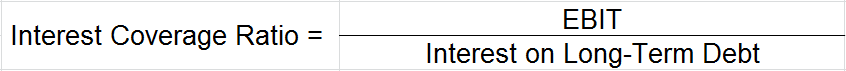# What is Interest Coverage Ratio?

## Interest Coverage Ratio

Interest Coverage ratio is a type of solvency ratio (long-term solvency) which is derived by dividing “Earnings before Interest and Taxes” of a company with its “Interest on Long-Term Debt“. Ideal number for this ratio is 1.5 or above, anything less than that shows the company doesn’t earn enough w.r.t its interest payments.

The ratio helps lenders and debenture holders to measure the capacity of a company to pay the interest liability on its long-term borrowings; higher the ratio greater the ability of the company to service its interest and hence lesser the risk of a financial default.

Interest Coverage ratio is also known as Times-Interest-Earned ratio.

## Formula to Calculate Interest Coverage RatioEBIT: Earnings before Interest and Taxes

Interest on Long-Term Debt:  Total interest obligation of the company on its long-term borrowings

## Explanation with an Example

From the below information of Unreal Corp. calculate its interest coverage ratio

Long Term-Loans = 1,00,000

Total Interest on Loans = 5,000

EBIT = 1,00,000

Interest Coverage Ratio = EBIT/Interest Expenses

= 1,00,000/5,000

= 20 (In this case the company’s earnings before interest and taxes are 20 times that of their interest expenses in an accounting period)

High Ratio – High coverage ratio depicts good financial condition & shows that the company earns enough profit before taxes and interest payments to pay off its interest expenses. The ability to pay interest obligations is critical for a company to stay in business as per going concern concept.

Low Ratio – Low coverage ratio shows the company is burdened by debt expenses and doesn’t earn enough EBIT relatively to its interest expenses. Banks and NBFCs would hesitate extending credit to such an organization.